College Chemistry : Gibb's Free Energy

Example Questions

Example Question #1 : Gibb's Free Energy

How do we know if a reaction is spontaneous?

The first law of thermodynamics

The sign of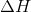The second law of thermodynamics

The value of(the rate constant) tells us

The sign of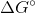The sign ofExplanation:

A reaction is spontaneous if and only if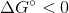. The sign ofonly tells us if a reaction is exothermic or endothermic. The rate constant only tells us the rate at which a given chemical reaction proceeds based on the reactants and the products. The first law of thermodynamics only tells us that energy is conserved, and can neither be created nor destroyed. The second law of thermodynamics states that the entropy of the universe is spontaneously increasing, however, under the right conditions, these reactions may proceed.

Example Question #1 : Thermodynamics And Kinetics

What is the standard change in free energy for a reaction run at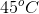with an equilibrium constant of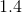?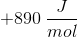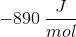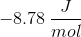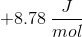Explanation:

In this question, we're told of a reaction that is run at a given temperature and with a certain equilibrium constant. We're asked to evaluate the standard change in free energy for this reaction.

First, right off the bat, we can see that the equilibrium constant for the reaction is greater than one. As a result, we know that under standard conditions, equilibrium of this reaction will favor products. Hence, we know right away that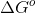will be negative, which allows us to rule out any answer choices that are positive.

To actually calculate the value of, we'll need to use an equation that relates this quantity with temperature and the equilibrium constant. We can do so by using the following expression.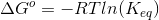If we plug in the values that we know, we can solve for the correct answer.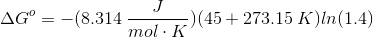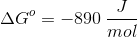Example Question #3 : Gibb's Free Energy

Calculate the change in free energy, in kilojoules, of the reaction at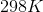by using the free energies of formation.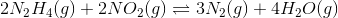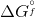values, in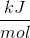, for molecules are as follows: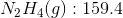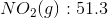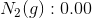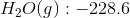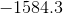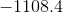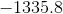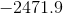Explanation:

Recall how to find the change in free energy for a reaction from using free energies of formation: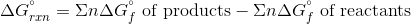Whereis the number of moles of the molecule.

Plug in the given values to find the change in free energy for the reaction.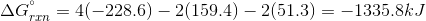Example Question #4 : Gibb's Free Energy

Suppose that a given chemical reaction has an enthalpy change of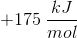and an entropy change of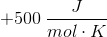. At what temperature would this chemical reaction be in equilibrium?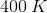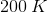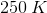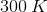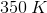Explanation:

For this question, we're asked to determine the temperature needed for a reaction to be in a state of equilibrium. We are also provided with the entropy and enthalpy changes for this reaction.

Remember that for a reaction to be in a state of equilibrium, the value for its change in free energy must be equal to zero. Furthermore, we can state the relationship between the change in free energy with the change in entropy, the change in enthalpy, and the temperature as follows.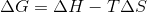Because the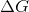term in the above expression must be equal to zero, we can plug this value in and then isolate the term for temperature.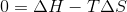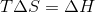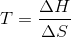Now that we have this expression, we just need to plug in the two values given to us in the question stem. Remember to make the units match though!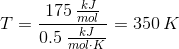All College Chemistry Resources